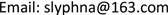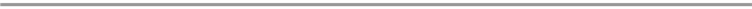﻿ RN上一类拟线性N-拉普拉斯方程的无穷解 Infinitely Many Solutions to a Class of Quasilinear N-Laplacian Equations in RN

Vol. 09  No. 03 ( 2020 ), Article ID: 34535 , 11 pages
10.12677/AAM.2020.93037

Infinitely Many Solutions to a Class of Quasilinear N-Laplacian Equations in ${ℝ}^{N}$

Guixiang Du, Jing Li

College of Mathematics and Statistics, Linyi University, Linyi ShandongReceived: Feb. 25th, 2020; accepted: Mar. 9th, 2020; published: Mar. 16th, 2020ABSTRACT

Under the assumption of the nonlinearity with critical exponential growth, we consider the existence of solutions to a class of quasilinear N-Laplacian equations in ${ℝ}^{N}$. By symmetric mountain pass lemma and variational argument, the existence of solutions is established.

Keywords:Trudinger-Moser Inequality, Symmetric Mountain Pass Lemma, Critical Exponential Growth${ℝ}^{N}$ 上一类拟线性N-拉普拉斯方程的无穷解1. 引言及主要结论

$-{\Delta }_{N}u+V\left(x\right){|u|}^{N-2}u-{\Delta }_{N}\left({|u|}^{2\alpha }\right){|u|}^{2\alpha -2}u=g\left(x\right),\text{\hspace{0.17em}}x\in {ℝ}^{N},$ (1.1)

( ${\mathcal{V}}_{1}$ ) 存在 ${V}_{0}>0$ 使得在 ${ℝ}^{N}$$V\left(x\right)\ge {V}_{0}$。此外 $V\left(x\right)\to \infty$$|x|\to \infty$ ；或者更为一般化对每个 $M>0$$\text{meas}\left(\left\{x\in {R}^{N}:V\left(x\right)\le M\right\}\right)<\infty$。“其中meas”为 ${ℝ}^{N}$ 上Lebesgue测度。

( ${\mathcal{F}}_{1}$ ) 奇函数 $g\left(s\right)\in {C}^{1}\left(ℝ\right)$$g\left(t\right)>0$$t>0$ 且存在 ${C}_{0}$${\alpha }_{0}$$q>0$ 使得

$|g\left(s\right)|\le {C}_{0}{|s|}^{q-1}\left[\mathrm{exp}\left({\alpha }_{0}{|s|}^{\frac{2\alpha N}{N-1}}\right)-{S}_{N-2}\left({\alpha }_{0},s\right)\right],\text{\hspace{0.17em}}\forall s\in ℝ,$ (1.2)

${S}_{N-2}\left({\alpha }_{0},s\right)={\sum }_{k=0}^{N-2}\frac{{\alpha }_{0}^{k}}{k!}{|s|}^{\frac{2\alpha N}{N-1}},\text{\hspace{0.17em}}\forall s\in ℝ.$ (1.3)

( ${\mathcal{F}}_{2}$ ) 存在 $q>N$${\mu }_{0}>0$ 使得

$0\le qG\left(s\right)\le sg\left(s\right)\le {\mu }_{0}{|s|}^{q}\mathrm{exp}\left({\alpha }_{0}{|s|}^{\frac{2\alpha N}{N-1}}\right)$ (1.4)

( ${\mathcal{F}}_{3}$ ) 存在 ${\mu }_{1}>0$$q>N$ 使得

$G\left(s\right)\ge {\mu }_{1}{|s|}^{q},\text{\hspace{0.17em}}\forall s\in ℝ.$ (1.5)

$-\text{Δ}u+V\left(x\right)u-\text{Δ}\left({|u|}^{2\alpha }\right){|u|}^{2\alpha -2}u=\lambda {|u|}^{p-1}u,\text{\hspace{0.17em}}x\in {ℝ}^{N}$ (1.6)

$E=\left\{u\in {W}^{1,n}\left({ℝ}^{N}\right):{\int }_{{ℝ}^{N}}\left({|\nabla u|}^{N}+V\left(x\right){|u|}^{N}\right)\text{d}x<\infty \right\}$

${‖u‖}_{E}={\left({\int }_{{ℝ}^{N}}\left({|\nabla u|}^{N}+V\left(x\right){|u|}^{N}\right)\text{d}x\right)}^{\frac{1}{N}}.$ (1.7)

2. 使用须知

${\int }_{\Omega }{\text{e}}^{\alpha {|u|}^{\frac{N}{N-1}}}\text{d}x<\infty ,\forall \alpha >0$

$\mathrm{sup}{‖u‖}_{{W}_{0}^{1,N}\text{Ω}}\le 1{\int }_{\Omega }{\text{e}}^{\alpha {|u|}^{\frac{N}{N-1}}}\text{d}x\le C,\forall \alpha \le {\alpha }_{N},$

${\int }_{{ℝ}^{N}}\left({\text{e}}^{\alpha {|u|}^{\frac{N}{N-1}}}-{S}_{N-2}\left(\alpha ,u\right)\right)\text{d}x<\infty ,\forall \alpha >0$

${\int }_{{ℝ}^{N}}\left({\text{e}}^{\alpha {|u|}^{\frac{N}{N-1}}}-{S}_{N-2}\left(\alpha ,u\right)\right)\text{d}x\le C,$

${\int }_{{ℝ}^{N}}{|u|}^{q}\left({\text{e}}^{\alpha {|u|}^{\frac{N}{N-1}}}-{S}_{N-2}\left(\alpha ,u\right)\right)\text{d}x<\infty ,\text{\hspace{0.17em}}\forall u\in {W}^{1,N}\left({ℝ}^{N}\right)$ (2.1)

${\int }_{{ℝ}^{N}}{|u|}^{q}\left({\text{e}}^{\alpha {|u|}^{\frac{N}{N-1}}}-{S}_{N-2}\left(\alpha ,u\right)\right)\text{d}x\le C{‖u‖}_{{W}^{1,N}}^{q}\left({ℝ}^{N}\right),$ (2.2)

${‖u‖}_{q}\le c\left(N,r\right){q}^{1-1/N}{‖\nabla u‖}_{N}^{1-\frac{r}{q}}{‖u‖}_{r}^{\frac{r}{q}}$. (2.3)

${q}^{1-1/N}$ 为最佳指数，特别的有，

$c\left(N,N\right)=\frac{1}{\sqrt{\pi }}{\left(\frac{\Gamma \left(\frac{N}{2}\right)\Gamma \left(2N\right)}{2\Gamma {\left(N\right)}^{2}}\right)}^{1/N}\equiv {d}_{N}.$ (2.4)

${‖u‖}_{q}\le {d}_{N}{q}^{1-1/N}{‖u‖}_{Y}.$

$I\left(u\right)=\frac{1}{N}{\int }_{{ℝ}^{N}}\left(1+{\left(2\alpha \right)}^{N-1}{|u|}^{\left(2\alpha -1\right)N}\right){|\nabla u|}^{N}\text{d}x+\frac{1}{N}{\int }_{{ℝ}^{N}}V\left(x\right){|u|}^{N}\text{d}x-{\int }_{{ℝ}^{N}}G\left(u\right)\text{d}x.$ (2.5)

${f}^{\prime }\left(t\right)={\left(1+{\left(2\alpha \right)}^{N-1}{|f\left(t\right)|}^{\left(2\alpha -1\right)N}\right)}^{-1/N},t\ge 0,f\left(0\right)=0$ (2.6)

$f\left(t\right)=-f\left(-t\right),t\in \left(-\infty ,0\right].$

( ${f}_{1}$ ) $f\in {C}^{2}$ 唯一确定且在 上可逆，

( ${f}_{2}$ ) $0<{f}^{\prime }\left(t\right)\le 1$$\forall t\in {ℝ}^{N}$

( ${f}_{3}$ ) $|f\left(t\right)|\le |t|$$\forall t\in {ℝ}^{N}$

( ${f}_{4}$ ) 当 $t\to 0$ 时， $\frac{f\left(t\right)}{t}\to 1$

( ${f}_{5}$ ) $|f\left(t\right)|\le {\left(2\alpha \right)}^{1/2\alpha N}{|t|}^{1/2\alpha },\forall t\in {ℝ}^{N}$

( ${f}_{6}$ ) $\frac{1}{2}f\left(t\right)\le \alpha t{f}^{\prime }\left(t\right)\le \alpha f\left(t\right),\forall t\in \left[0,\infty \right)$ 以及 $\alpha f\left(t\right)\le \alpha t{f}^{\prime }\left(t\right)\le \frac{1}{2}f\left(t\right),\forall t\in \left(-\infty ,0\right]$

( ${f}_{7}$ ) $\exists a\in \left(0,{\left(2\alpha \right)}^{1/2\alpha N}\right)$ 使得 $\frac{f\left(t\right)}{{t}^{1/2\alpha }}\to a,t\to +\infty$

( ${f}_{8}$ ) $\exists {b}_{0}>0$ 使得

$|f\left(t\right)|\ge \left\{\begin{array}{l}{b}_{0}|t|\text{\hspace{0.17em}}\text{\hspace{0.17em}}\text{\hspace{0.17em}}\text{\hspace{0.17em}}\text{\hspace{0.17em}}\text{\hspace{0.17em}}\text{\hspace{0.17em}}\text{\hspace{0.17em}}|t|\le 1,\\ {b}_{0}{|t|}^{1/2\alpha }\text{\hspace{0.17em}}\text{\hspace{0.17em}}\text{ }\text{\hspace{0.17em}}|t|\ge 1.\end{array}$

$J\left(v\right)\equiv I\left(f\left(v\right)\right)=\frac{1}{N}{\int }_{{ℝ}^{N}}{|\nabla v|}^{N}\text{d}x+\frac{1}{N}{\int }_{{ℝ}^{N}}V\left(x\right){|f\left(v\right)|}^{N}\text{d}x-{\int }_{{ℝ}^{N}}G\left(f\left(v\right)\right)\text{d}x$ (2.7)

$v\in {W}^{1,N}\left({ℝ}^{N}\right)\cap {L}_{loc}^{\infty }\left({ℝ}^{N}\right)\left(p>1\right)$ 为泛函J的临界点，即对所有的 $\phi \in {W}^{1,N}\left({ℝ}^{N}\right)$${J}^{\prime }\left(v\right)\phi =0$ 其中

${J}^{\prime }\left(v\right)\phi ={\int }_{{R}^{N}}{|\nabla v|}^{N-2}\nabla v\nabla \phi \text{d}x+{\int }_{{R}^{N}}V\left(x\right){|f\left(v\right)|}^{N-2}f\left(v\right){f}^{\prime }\left(v\right)\phi \text{d}x-{\int }_{{R}^{N}}g\left(f\left(v\right)\right){f}^{\prime }\left(v\right)\phi \text{d}x$

$-{\Delta }_{N}v=h\left(v\right),x\in {ℝ}^{N},$ (2.8)

$|{\phi }_{n}\left(x\right)|\le 2\alpha |{v}_{n}\left(x\right)|,\text{\hspace{0.17em}}|\nabla {\phi }_{n}\left(x\right)|\le 2|\nabla {v}_{n}\left(x\right)|,\text{\hspace{0.17em}}{‖{\phi }_{n}\left(x\right)‖}_{E}\le 2\alpha {‖{v}_{n}\left(x\right)‖}_{E},\text{\hspace{0.17em}}\forall n\in ℕ$

$\begin{array}{c}c\ge J\left({v}_{n}\right)-\frac{1}{q}J\left({v}_{n}\right){v}_{n}\\ \ge \left(\frac{1}{N}-\frac{2\alpha }{q}\right){\int }_{{ℝ}^{N}}{|\nabla {v}_{n}|}^{N}\text{d}x+\left(\frac{1}{N}-\frac{1}{q}\right){\int }_{{ℝ}^{N}}V\left(x\right){|f\left(v\right)|}^{N}\text{d}x\\ \text{\hspace{0.17em}}\text{\hspace{0.17em}}+\frac{1}{q}{\int }_{{ℝ}^{N}}\left[g\left(f\left({v}_{n}\right)\right)f\left({v}_{n}\right)-qG\left(f\left({v}_{n}\right)\right)\right]\text{d}x\\ \ge \left(\frac{1}{N}-\frac{2\alpha }{q}\right){‖\nabla {v}_{n}‖}_{N}^{N}+\left(\frac{1}{N}-\frac{1}{q}\right){\int }_{{ℝ}^{N}}V\left(x\right){|f\left(v\right)|}^{N}\text{d}x\end{array}$ (2.9)

$q>2\alpha N>N$ 可推出序列 $\left\{{‖\nabla {v}_{n}‖}_{N}\right\}$ 以及 $\left\{{\int }_{{ℝ}^{N}}V\left(x\right){|f\left({v}_{n}\right)|}^{N}\right\}$ 有界，亦如此，其中

${A}_{n}^{N}={\int }_{{ℝ}^{N}}\left({|\nabla {v}_{n}|}^{N}+V\left(x\right){|f\left({v}_{n}\right)|}^{N}\right)\text{d}x$ (2.10)

${\int }_{{ℝ}^{N}}{|{v}_{n}|}^{N}\text{d}x={\int }_{‖{v}_{n}‖\le 1}{|{v}_{n}|}^{N}\text{d}x+{\int }_{‖{v}_{n}‖>1}{|{v}_{n}|}^{N}\text{d}x$ (2.11)

${\int }_{‖{v}_{n}‖>1}{|{v}_{n}|}^{N}\text{d}x\le \frac{1}{C}{\int }_{‖{v}_{n}‖>1}G\left(f\left({v}_{n}\right)\right)\text{d}x\le \frac{1}{C}{\int }_{{ℝ}^{N}}G\left(f\left({v}_{n}\right)\right)\text{d}x$

${\int }_{‖{v}_{n}‖\le 1}{|{v}_{n}|}^{N}\text{d}x\le \frac{1}{C}{\int }_{‖{v}_{n}‖\le 1}{|f\left({v}_{n}\right)|}^{N}\text{d}x\le \frac{1}{C}{\int }_{{ℝ}^{N}}{\int }_{‖{v}_{n}‖\le 1}{|f\left({v}_{n}\right)|}^{N}\text{d}x$

${\int }_{{ℝ}^{N}}{|\nabla {v}_{n}|}^{N}+V\left(x\right){|f\left({v}_{n}\right)|}^{N}\text{d}x\ge {C}_{0}{‖{v}_{n}‖}_{E}^{N}$ (2.12)

${v}_{n}⇀v\text{\hspace{0.17em}}\text{ }\text{in}\text{\hspace{0.17em}}E,\text{\hspace{0.17em}}{v}_{n}⇀v\text{\hspace{0.17em}}\text{in}\text{\hspace{0.17em}}{L}_{loc}^{q}\left({ℝ}^{N}\right),\forall q\in \left[N,+\infty \right),{v}_{n}\left(x\right)⇀v\left(x\right)\text{\hspace{0.17em}}a.e.\text{\hspace{0.17em}}\text{in}\text{\hspace{0.17em}}{ℝ}^{N}$ (2.13)

$\underset{n\to \infty }{\mathrm{lim}}{\int }_{{R}^{N}}g\left(f\left({v}_{n}\right)\right){f}^{\prime }\left({v}_{n}\right){v}_{n}\text{d}x={\int }_{{R}^{N}}g\left(f\left(v\right)\right){f}^{\prime }\left(v\right)v\text{d}x$ (2.14)

$|G\left(s\right)|\le \epsilon \left({|s|}^{N}+sg\left(s\right)\right)+{\chi }_{\left[{s}_{0},{S}_{0}\right]}\left(|s|\right)|sg\left(s\right)|,\forall s\in ℝ$ (2.15)

$|g\left(f\left({v}_{n}\right)\right){f}^{\prime }\left({v}_{n}\right){v}_{n}|\le |g\left(f\left({v}_{n}\right)\right)f\left({v}_{n}\right)|\le \epsilon \left(V{|f\left({v}_{n}\right)|}^{N}+f\left({v}_{n}\right)g\left(f\left({v}_{n}\right)\right)\right)+{\chi }_{\left[{s}_{0},{S}_{0}\right]}\left(|f\left({v}_{n}\right)|\right)|f\left({v}_{n}\right)g\left(f\left({v}_{n}\right)\right)|$,

${\int }_{{B}_{r}^{c}}|g\left(f\left({v}_{n}\right)\right){f}^{\prime }\left({v}_{n}\right){v}_{n}|\le \epsilon |Q\left({v}_{n}\right)|+{S}_{0}g\left({S}_{0}\right){\int }_{{A}_{n}\cap {B}_{r}^{c}}{\chi }_{\left[{s}_{0},{S}_{0}\right]}\left(|f\left({v}_{n}\right)|\right)\text{d}x$ (2.16)

$Q\left({v}_{n}\right)={\int }_{{ℝ}^{N}}\left(V\left(x\right){|f\left({v}_{n}\right)|}^{N}+f\left({v}_{n}\right)g\left(f\left({v}_{n}\right)\right)\right)\text{d}x$

${s}_{0}g\left({s}_{0}\right)|{A}_{n}|\le {\int }_{{ℝ}^{N}}f\left({v}_{n}\right)g\left(f\left({v}_{n}\right)\right)\text{d}x\le {M}_{1}$

$\underset{r\to \infty }{\mathrm{lim}}|{A}_{n}\cap {B}_{r}^{c}|=0$ (2.17)

$|{A}_{{n}_{0}}\cap {B}_{{r}_{j}}^{c}|\ge \delta ,\forall j\in N$

${\Omega }_{j}={B}_{{r}_{j}}^{c}\\stackrel{¯}{{B}_{{r}_{j+1}}^{c}}$，易见

${B}_{{r}_{j}}^{c}=\underset{l=j}{\overset{\infty }{\cup }}{\Omega }_{l},\forall j\in N,{\Omega }_{l}\cap {\Omega }_{k}=\varnothing ,l\ne k$

$|{A}_{1}\cap {B}_{{r}_{j}}^{c}|={\sum }_{l=j}^{\infty }|{A}_{1}\cap {\Omega }_{l}|\ge \delta ,\forall j\in N$ (2.18)

${\int }_{{B}_{r}^{c}}{|v|}^{N}\text{d}x\le ϵ$ (2.19)

${\int }_{{D}_{j}}{|v|}^{N}\text{d}x\le \frac{ϵ}{{2}^{j}},\text{\hspace{0.17em}}\forall j\in N$ (2.20)

$\underset{n\to \infty }{\mathrm{lim}}\mathrm{sup}{\int }_{{D}_{j}\cap {A}_{n}}{|f\left({v}_{n}\right)|}^{N}\text{d}x\le {\int }_{{D}_{j}}\underset{n\to \infty }{\mathrm{lim}}\mathrm{sup}{|f\left({v}_{n}\right)|}^{N}\text{d}x\le {\int }_{{D}_{j}}{|f\left(v\right)|}^{N}\text{d}x\le \frac{ϵ}{{2}^{j}}$ (2.21)

$\begin{array}{c}{s}_{0}^{N}\underset{n\to \infty }{\mathrm{lim}}\mathrm{sup}|{A}_{n}\cap {B}_{{r}_{0}}^{c}|\le \underset{n\to \infty }{\mathrm{lim}}\underset{n\to \infty }{\mathrm{lim}}\mathrm{sup}{\int }_{{B}_{{r}_{0}}^{c}\cap {A}_{n}}{|f\left({v}_{n}\right)|}^{N}\text{d}x\\ =\underset{n\to \infty }{\mathrm{lim}}\mathrm{sup}{\sum }_{j=1}^{\infty }{\int }_{{D}_{j}\cap {A}_{n}}{|f\left({v}_{n}\right)|}^{N}\text{d}x\\ \le {\sum }_{j=1}^{\infty }\underset{n\to \infty }{\mathrm{lim}}\mathrm{sup}{\int }_{{D}_{j}\cap {A}_{n}}{|f\left({v}_{n}\right)|}^{N}\text{d}x\\ \le {\sum }_{j=1}^{\infty }{\int }_{{D}_{j}}{|f\left({v}_{n}\right)|}^{N}\text{d}x\le {\sum }_{j=1}^{\infty }\frac{ϵ}{{2}^{j}}=ϵ\end{array}$ (2.22)

${\int }_{{A}_{n}\cap {B}_{r}^{c}}{\chi }_{\left[{s}_{0},{S}_{0}\right]}\left(|f\left({v}_{n}\right)|\right)\text{d}x<\frac{ϵ}{{S}_{0}g\left({S}_{0}\right)}$ (2.23)

${\int }_{{B}_{r}^{c}}|g\left(f\left({v}_{n}\right)\right){f}^{\prime }\left({v}_{n}\right){v}_{n}|\text{d}x\le {\int }_{{B}_{r}^{c}}|g\left(f\left({v}_{n}\right)\right)f\left({v}_{n}\right)|\text{d}x\le ϵ\left({M}_{2}+1\right),r\ge {r}_{0}$ (2.24)

${\int }_{{B}_{r}^{c}}|g\left(f\left(v\right)\right){f}^{\prime }\left(v\right)v|\text{d}x\le {\int }_{{B}_{r}^{c}}|g\left(f\left(v\right)\right)f\left(v\right)|\text{d}x\le {\int }_{{B}_{r}^{c}}|g\left(f\left({v}_{n}\right)\right)f\left({v}_{n}\right)|\text{d}x\le ϵ\left({M}_{2}+1\right),r\ge {r}_{0}$ (2.25)

$\underset{n\to \infty }{\mathrm{lim}}{\int }_{{B}_{r}}g\left(f\left({v}_{n}\right)\right){f}^{\prime }\left({v}_{n}\right){v}_{n}\text{d}x={\int }_{{B}_{r}}g\left(f\left(v\right)\right){f}^{\prime }\left(v\right)v\text{d}x$ (2.26)

$\underset{n\to \infty }{\mathrm{lim}}\mathrm{sup}{\int }_{{ℝ}^{N}}V\left(x\right){|f\left({v}_{n}\right)|}^{N}\text{d}x={\int }_{{ℝ}^{N}}V\left(x\right){|f\left(v\right)|}^{N}\text{d}x$ (2.27)

$\underset{n\to \infty }{\mathrm{lim}}{\int }_{{ℝ}^{N}}V\left(x\right){|f\left({v}_{n}\right)|}^{N-2}f\left({v}_{n}\right){f}^{\prime }\left({v}_{n}\right){v}_{n}\text{d}x={\int }_{{ℝ}^{N}}V\left(x\right){|f\left(v\right)|}^{N}f\left(v\right){f}^{\prime }\left(v\right)v\text{d}x$ (2.28)

${\eta }_{r}\left(|x|\right)\equiv 1,x\in {B}_{2r}^{c};\text{\hspace{0.17em}}{\eta }_{r}\left(|x|\right)=0,x\in {B}_{r};0\le {\eta }_{r}\le 1,|\nabla {\eta }_{r}|\le \frac{2}{r},\text{\hspace{0.17em}}\text{in}\text{\hspace{0.17em}}{ℝ}^{N}$ (2.29)

$\begin{array}{l}{\int }_{{ℝ}^{N}}{|\nabla {v}_{n}|}^{N-2}\nabla {v}_{n}\nabla {\phi }_{n}{\eta }_{r}\text{d}x+{\int }_{{ℝ}^{N}}V\left(x\right){|f\left({v}_{n}\right)|}^{N}{\eta }_{r}\text{d}x\\ =-{\int }_{{ℝ}^{N}}{|\nabla {v}_{n}|}^{N-2}\nabla {v}_{n}\nabla {\eta }_{r}{\phi }_{n}\text{d}x+{\int }_{{ℝ}^{N}}g\left(f\left({v}_{n}\right)\right)f\left({v}_{n}\right){\eta }_{r}\text{d}x+{o}_{n}\left(1\right)\end{array}$ (2.30)

$\begin{array}{l}{\int }_{{B}_{r}^{c}}\left({|\nabla {v}_{n}|}^{N}+V\left(x\right){|f\left({v}_{n}\right)|}^{N}\right){\eta }_{r}\text{d}x\\ \le {\int }_{{B}_{r}^{c}}{|\nabla {v}_{n}|}^{N-2}|{v}_{n}||\nabla {\eta }_{r}|\text{d}x+{o}_{n}\left(1\right)+\omega \left(r\right)\\ \le \frac{4\alpha }{r}{\int }_{{B}_{r}^{c}}{|\nabla {v}_{n}|}^{N-2}|{v}_{n}|\text{d}x+{o}_{n}\left(1\right)+\omega \left(r\right)\\ \le \frac{4\alpha }{r}{‖\nabla {v}_{n}‖}_{{L}^{N}\left({\Omega }_{r}\right)}^{N-1}{‖{v}_{n}‖}_{{L}^{N}\left({\Omega }_{r}\right)}+{o}_{n}\left(1\right)+\omega \left(r\right)\\ \le \frac{4\alpha }{r}{‖{v}_{n}‖}_{E}^{N}+{o}_{n}\left(1\right)+\omega \left(r\right)\\ \le \frac{4\alpha }{r}{M}^{p}+{o}_{n}\left(1\right)+\omega \left(r\right),n\to \infty ,r\to \infty \end{array}$ (2.31)

${\mathrm{lim}}_{n\to \infty }\mathrm{sup}{\int }_{{B}_{2r}^{c}}\left(V\left(x\right){|f\left({v}_{n}\right)|}^{N}\right)\text{d}x\le ϵ$ (2.32)

${\int }_{{B}_{2r}^{c}}\left(V\left(x\right){|f\left(v\right)|}^{N}\right)\text{d}x\le ϵ$ (2.33)

${\mathrm{lim}}_{n\to \infty }{\int }_{{B}_{2r}}V\left(x\right){|f\left({v}_{n}\right)|}^{N}\text{d}x={\int }_{{B}_{2r}}V\left(x\right){|f\left(v\right)|}^{N}\text{d}x$ (2.34)

$\forall ϵ>0$，极限(2.32)~(2.34)产生

${\mathrm{lim}}_{n\to \infty }\mathrm{sup}{\int }_{{R}^{N}}|V\left(x\right)\left({|f\left({v}_{n}\right)|}^{N}-{|f\left(v\right)|}^{N}\right)\text{d}x|\le 3ϵ$. (2.35)

${\int }_{{ℝ}^{N}}g\left(f\left({v}_{n}\right)\right){f}^{\prime }\left({v}_{n}\right){v}_{n}{\eta }_{r}\text{d}x\le {\int }_{{ℝ}^{N}}g\left(f\left({v}_{n}\right)\right)f\left({v}_{n}\right){\eta }_{r}\text{d}x=\omega \left(r\right)\to 0$ (2.36)

$\begin{array}{l}{\int }_{{ℝ}^{N}}\left[{|\nabla {v}_{n}|}^{N}+V\left(x\right){|f\left({v}_{n}\right)|}^{N-2}f\left({v}_{n}\right){f}^{\prime }\left({v}_{n}\right)\right]{\eta }_{r}\text{d}x\\ =-{\int }_{{ℝ}^{N}}{|\nabla {v}_{n}|}^{N-2}\nabla {v}_{n}\nabla {\eta }_{r}{v}_{n}\text{d}x+{\int }_{{ℝ}^{N}}g\left(f\left({v}_{n}\right)\right){f}^{\prime }\left({v}_{n}\right){\eta }_{r}\text{d}x+{o}_{n}\left(1\right)\\ \le {\int }_{{ℝ}^{N}}{|\nabla {v}_{n}|}^{N-1}|\nabla {v}_{n}||\nabla {\eta }_{r}|\text{d}x+{o}_{n}\left(1\right)+\omega \left(r\right)\\ \le \frac{4\alpha }{r}{M}^{p}+{o}_{n}\left(1\right)+\omega \left(r\right)\end{array}$

${\mathrm{lim}}_{n\to \infty }\mathrm{sup}{\int }_{{B}_{2r}^{c}}\left[{|\nabla {v}_{n}|}^{N}{\eta }_{r}+V\left(x\right){|f\left({v}_{n}\right)|}^{N-2}f\left({v}_{n}\right){f}^{\prime }\left({v}_{n}\right){v}_{n}\right]\text{d}x\le ϵ$ (2.37)

${\mathrm{lim}}_{n\to \infty }\mathrm{sup}{\int }_{{B}_{2r}^{c}}V\left(x\right){|f\left({v}_{n}\right)|}^{N-2}f\left({v}_{n}\right){f}^{\prime }\left({v}_{n}\right){v}_{n}\text{d}x\le ϵ$ (2.38)

${\int }_{{B}_{2r}^{c}}V\left(x\right){|f\left(v\right)|}^{N-2}f\left({v}_{n}\right){f}^{\prime }\left(v\right)v\text{d}x\le ϵ$ (2.39)

${\mathrm{lim}}_{n\to \infty }\mathrm{sup}{\int }_{{B}_{2r}}V\left(x\right){|f\left({v}_{n}\right)|}^{N-2}f\left({v}_{n}\right){f}^{\prime }\left({v}_{n}\right){v}_{n}\text{d}x={\int }_{{B}_{2r}}V\left(x\right){|f\left(v\right)|}^{N-2}f\left({v}_{n}\right){f}^{\prime }\left(v\right)v\text{d}x$

$\left({|\xi |}^{p-2}\xi -{|\eta |}^{p-2}\eta ,\xi -\eta \right)\ge {C}_{3}{\left(|\zeta |+|\eta |\right)}^{p-2}{|\xi -\eta |}^{2},\text{\hspace{0.17em}}\text{if}\text{\hspace{0.17em}}1

$\left({|\xi |}^{p-2}\xi -{|\eta |}^{p-2}\eta ,\xi -\eta \right)\ge {C}_{3}{|\xi -\eta |}^{p},\text{\hspace{0.17em}}\text{if}\text{\hspace{0.17em}}p\ge 2$

${B}_{nm}={\int }_{{R}^{N}}V\left(x\right)\left[{|f\left({v}_{n}\right)|}^{N-2}f\left({v}_{n}\right){f}^{\prime }\left({v}_{n}\right){v}_{n}-{|f\left(v\right)|}^{N-2}f\left(v\right){f}^{\prime }\left(v\right)v\right]\left({v}_{n}-v\right)\text{d}x$

${C}_{nm}={\int }_{{ℝ}^{N}}\left[g\left(f\left({v}_{n}\right)\right){f}^{\prime }\left({v}_{n}\right)d-g\left(f\left(v\right)\right)f\left(v\right)\right]\left({v}_{n}-v\right)\text{d}x$

${A}_{nm}\ge {\int }_{{ℝ}^{N}}\left({|\nabla \left({v}_{n}-v\right)|}^{N}\right)\text{d}x\to 0.$

$|f{\left({v}_{n}-v\right)}^{N}|\le {|{v}_{n}-v|}^{N}$ 以及在 ${L}^{N}\left({ℝ}^{N}\right)$${v}_{n}\to v$，可推出 ${\int }_{{ℝ}^{N}}V\left(x\right){|f\left({v}_{n}-v\right)|}^{N}\text{d}x\to 0,n\to \infty$，于是在E中 ${v}_{n}\to v$

3. 定理证明

( ${J}_{1}$ ) $\exists \rho ,\alpha >0$ 使得在 $\partial {B}_{\rho \cap V}$ 上有 $J\left(u,v\right)\ge \alpha$

( ${J}_{2}$ ) 对任一有限维子空间 ${E}_{0}\subset E$，有 $R=R\left({E}_{0}\right)$ 使得在 ${E}_{0}\{B}_{R}$ 上有 $J\left(u,v\right)\le 0$，其中 ${B}_{R}=\left\{z\in E:{|z|}_{E}

$J\left(v\right)=\frac{1}{N}{\int }_{{ℝ}^{N}}{|\nabla v|}^{N}\text{d}x+\frac{1}{N}{\int }_{{ℝ}^{N}}V\left(x\right){|f\left(v\right)|}^{N}\text{d}x-{\int }_{{ℝ}^{N}}G\left(f\left(v\right)\right)\text{d}x$, (3.1)

${\int }_{{ℝ}^{N}}\left({|\nabla v|}^{N}+V\left(x\right){|f\left(v\right)|}^{N}\right)\text{d}x\ge {C}_{0}{‖v‖}_{E}^{N}$

$G\left(t\right)\le \frac{ϵ}{N}{|t|}^{N}+{C}_{ϵ}tg\left(t\right)$

$\begin{array}{l}0\le {\int }_{{ℝ}^{N}}g\left(f\left(v\right)\right)f\left(v\right)\text{d}x\\ \le {b}_{1}{\int }_{{ℝ}^{N}}{|f\left(v\right)|}^{q}\left[\mathrm{exp}\left({\alpha }_{0}{|f\left(v\right)|}^{\frac{2\alpha N}{N-1}}\right)-{S}_{N-2}\left({\alpha }_{0},f\left(v\right)\right)\right]\text{d}x\\ \le {\sum }_{k=N-1}^{\infty }\frac{{b}_{1}{\alpha }_{0}^{k}}{k!}{\int }_{{ℝ}^{N}}{|f\left(v\right)|}^{q-\frac{2\alpha Nk}{N-1}}\text{d}x\le {\sum }_{k=N-1}^{\infty }\frac{{b}_{2}{\alpha }_{0}^{k}}{k!}{\left(2\alpha \right)}^{\frac{k}{N-1}}{\int }_{{ℝ}^{N}}{|v|}^{{q}_{k}+{q}_{0}}\text{d}x\\ \le {b}_{2}{\sum }_{k=N-1}^{\infty }\frac{{\alpha }_{0}^{k}}{k!}{\left(2\alpha \right)}^{\frac{k}{N-1}}{d}_{N}^{{q}_{k}+{q}_{0}}{\left({q}_{k}+{q}_{0}\right)}^{\left(1-\frac{1}{N}\right)\left({q}_{k}+{q}_{0}\right)}{‖v‖}_{E}^{{q}_{k}+{q}_{0}}\\ \le {b}_{2}{d}_{N}^{{q}_{0}}{‖v‖}_{E}^{{q}_{0}}{\sum }_{k=N-1}^{\infty }{a}_{k}\end{array}$, (3.2)

${b}_{2}={b}_{1}{\left(2\alpha \right)}^{\frac{q}{2\alpha N}},{q}_{o}=\frac{q}{2\alpha },\beta =\frac{N+{q}_{0}}{N-1},{q}_{k}=\frac{kN}{N-1},{a}_{k}=\frac{{\left({\left(2\alpha \right)}^{\frac{1}{N-1}}{\alpha }_{0}\right)}^{k}}{k!}{d}_{N}^{{q}_{k}}{‖v‖}_{E}^{{q}_{k}}{\left(\beta k\right)}^{k+{q}_{0}\left(1-\frac{1}{N}\right)}.$

${\mathrm{lim}}_{k\to \infty }\frac{{a}_{k+1}}{{a}_{k}}=e{\alpha }_{0}\beta {\left(2\alpha \right)}^{\frac{1}{N-1}}{‖v‖}_{E}^{\frac{N}{N-1}}{d}_{N}^{\frac{N}{N-1}}\le e{\alpha }_{0}\beta {\left(2\alpha \right)}^{\frac{1}{N-1}}{\rho }^{\frac{N}{N-1}}{d}_{N}^{\frac{N}{N-1}}<1.$ (3.3)

$0\le {\int }_{{ℝ}^{N}}g\left(f\left(v\right)\right)f\left(v\right)\text{d}x\le {C}_{1}{‖v‖}_{E}^{{q}_{0}}.$

$J\left(v\right)\ge \frac{{C}_{0}-ϵ}{N}{‖v‖}_{E}^{N}-{C}_{1}{‖v‖}_{E}^{{q}_{0}}$ (3.4)

$\frac{1}{N}{\int }_{{ℝ}^{N}}\left({|\nabla v|}^{N}+{\int }_{{ℝ}^{N}}V\left(x\right){|f\left(v\right)|}^{N}\right)\text{d}x\ge {\int }_{{ℝ}^{N}}G\left(f\left(v\right)\right)\text{d}x\ge {C}_{2}{\int }_{{ℝ}^{N}}{|f\left(v\right)|}^{q}\text{d}x$ (3.5)

${\omega }_{n}\left(x\right)=\frac{{v}_{n}\left(x\right)}{‖{v}_{n}\left(x\right)‖}$。假设在E中 ${\omega }_{n}⇀\omega ,{\omega }_{n}\left(x\right)⇀\omega \left(x\right)\text{\hspace{0.17em}}a.e.\text{\hspace{0.17em}}\text{in}\text{\hspace{0.17em}}{ℝ}^{N}$。记 $\Omega =\left\{x\in {ℝ}^{N}:\omega \left(x\right)\ne 0\right\}$。假定 $|\Omega |>0$$\Omega$ 中显然有 ${v}_{n}\left(x\right)\to \infty$，那么有

$\begin{array}{c}\frac{1}{N}{v}_{E}^{N}=\frac{1}{N}{\int }_{{ℝ}^{N}}\left({|\nabla v|}^{N}+{\int }_{{ℝ}^{N}}V\left(x\right){|v|}^{N}\right)\text{d}x\\ \ge \frac{1}{N}{\int }_{{ℝ}^{N}}\left({|\nabla v|}^{N}+{\int }_{{ℝ}^{N}}V\left(x\right){|f\left(v\right)|}^{N}\right)\text{d}x\\ \ge {C}_{2}{\int }_{{ℝ}^{N}}{|f\left(v\right)|}^{q}\text{d}x\end{array}$

$\frac{1}{N}\ge {C}_{2}{\int }_{{ℝ}^{N}}\frac{{|f\left({v}_{n}\right)|}^{q}}{{|{v}_{n}|}^{N}}{\omega }_{n}^{E}\text{d}x\to \infty ,n\to \infty$ (3.6)

${\left({\int }_{{ℝ}^{N}}{|v|}^{q}\text{d}x\right)}^{\frac{1}{q}}\ge \theta {v}_{E},\forall v\in {E}_{0},{\int }_{{ℝ}^{N}}{|v|}^{q}\text{d}{x}^{\frac{1}{q}}\ge {\theta }^{q}{‖{v}_{n}‖}_{E}^{q}$ (3.7)

Infinitely Many Solutions to a Class of Quasilinear N-Laplacian Equations in RN[J]. 应用数学进展, 2020, 09(03): 307-317. https://doi.org/10.12677/AAM.2020.93037

1. 1. Adachi, S. and Watanabe, T. (2012) Uniqueness of the Ground State Solutions of Quasilinear Schr?dinger Equations. Nonlinear Analysis, 75, 819-833. https://doi.org/10.1016/j.na.2011.09.015

2. 2. Colin, M. and Jeanjean, L. (2004) Solutions for a Quasilinear Schr?dinger Equation: A Dual Approach. Nonlinear Analysis, 56, 213-226. https://doi.org/10.1016/j.na.2003.09.008

3. 3. Popenberg, M., Schmitt, K. and Wang, Z.Q. (2002) On the Existence of Soliton Solutions to Quasilinear Schr?dinger Equations. Calculus of Variations and Partial Differential Equations, 14, 329-344. https://doi.org/10.1007/s005260100105

4. 4. Liu, J., Wang, Y. and Wang, Z.Q. (2004) Solutions for Quasilinear Schr?dinger Equations via Nehari Method. Communications in Partial Differential Equations, 29, 879-901. https://doi.org/10.1081/PDE-120037335

5. 5. Do ó, J.M.B. (1997) N-Laplacian Equations in RN with Critical Growth. Abstract and Applied Analysis, 2, 301-315.https://doi.org/10.1155/S1085337597000419

6. 6. Liu, J. and Wang, Z.Q. (2002) Soliton Solutions for Quasilinear Schr?dinger Equations I. Proceedings of the American Mathematical Society, 131, 441-448. https://doi.org/10.1090/S0002-9939-02-06783-7

7. 7. Liu, J., Wang, Y. and Wang, Z. (2003) Soliton Solutions for Quasilinear Schr?dingerr Equations II. Proceedings of the American Mathematical Society, 187, 473-493. https://doi.org/10.1016/S0022-0396(02)00064-5

8. 8. Chen, C.S. and Zhu, Q. (2014) Existence of Positive Solu-tions to p-Kirchhoff-Type Problem without Compactness Conditions. Applied Mathematics Letters, 28, 82-87. https://doi.org/10.1016/j.aml.2013.10.005

9. 9. Trudinger, N. (1967) On Imbeddding into Orlicz Space and Some Applications. Journal of Mathematics and Mechanics, 17, 473-484. https://doi.org/10.1512/iumj.1968.17.17028

10. 10. Moser, J. (1971) A Sharp Form of an Inequality by N. Trudinger. Indiana University Mathematics Journal, 20, 1077-1092. https://doi.org/10.1512/iumj.1971.20.20101

11. 11. Cao, D.M. (1992) Nontrivial Solution of a Semilinear Elliptic Equation with Critical Exponent in R2. Communications in Partial Differential Equations, 17, 407-435. https://doi.org/10.1080/03605309208820848

12. 12. Kosevich, A.M., Ivanov, B.A. and Kovalev, A.S. (1990) Magnetic Solitons. Physics Reports, 194, 117-238.https://doi.org/10.1016/0370-1573(90)90130-T

13. 13. Dinca, G. and Jebelean, P. (2001) Some Existence Results for a Class of Nonlinear Equations Involving a Duality Mapping. Nonlinear Analysis, 46, 347-363. https://doi.org/10.1016/S0362-546X(00)00120-6

14. 14. Rabinowitz, P.H. (1986) Minimax Methods in Critical Point Theory with Application to Differential Equations. In: CBMS Regional Conference Series in Mathematics, Volume 65, American Mathematical Society, Providence, RI.https://doi.org/10.1090/cbms/065